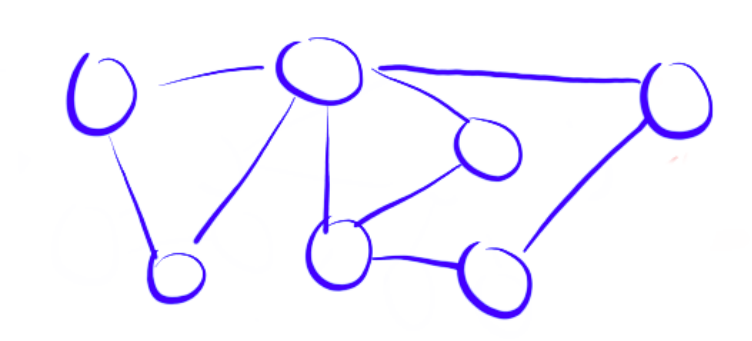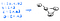# Algorithms on Graphs: What is a graph?

To simply put it, a graph is a collection of vertices and a collection of edges such that each edge connects a pair of vertices together. A visual representation would be:the nodes (vertices) are connected by the lines (edges)

However, graphs can come with various properties and varying sizes. Here are a few examples:

At this moment, we’re not going to delve into all these examples right away. With ease, we’re going to learn much about the varying types of graphs and the algorithms that are used for them.

While it’s nice to see how the graphs are represented in a visual sense, let’s talk about how they are often represented in code.

# Graph Representation

Let’s take this graph as an example and see how we would represent it in code.

## Adjacency Matrix

One way it could be represented would be by an adjacency matrix that looks like this.

Notice how this is a n x n matrix that’s symmetric along the diagonal. The symmetry is really due to the nature of our example. Since our graph is bidirectional, it makes sense that our matrix is symmetric since both vertices are neighbors with each other.

Now, we know that often when we’re dealing with n x n matrices, it’s most often represented as a 2D array like this:

Now this is great if we’re trying to find a value at (i,j) because an index lookup on an array is O(1) (constant time).

However, having an array representation does come with some cons. O(1) access to nodes is nice if we already know which nodes to look for.

However, when we’re traversing through a node’s adjacent neighbors, we have to look at each node to determine if it’s a neighbor which will take O(V) time, where V is the number of nodes in the graph.

Not only that, but notice how we have a bunch of 0’s in the array to indicate that a node is not neighbors with another node. These 0’s can unnecessarily take up a lot of space and make our memory O(V²).

Let’s look at another representation that we can use.

## Adjacency List

Another way we can condense space and search time of neighbors is through an adjacency list:We could represent this using a hashmap or an array that contains ArrayList as elements

The adjacency list allows us to keep track of only neighboring nodes. This allows us to condense our memory usage from O(V²) to O(V + E), where E is the number of edges in the graph.

Lookup time for a node’s neighbors are also shortened to O(E) because E represents the number of edges connected to the node (or another way to see it is that E represents the number of neighbors that the node has).

Throughout much of these posts, I’ll be using the adjacency list over the adjacency matrix in order to optimize our algorithms.

# Summary

• Graphs come in varying size and shape. We’ll discuss how they influence our algorithms in future posts.
• A graph can be represented either as an adjacency matrix or an adjacency list.
• An adjacency matrix allows us O(1) access if we know the node but take up O(V) times for searching neighbors and O(V²) space.
• An adjacency list cuts our search time to O(E) and our memory to O(V + E).

## More from Try Khov

Software Engineer